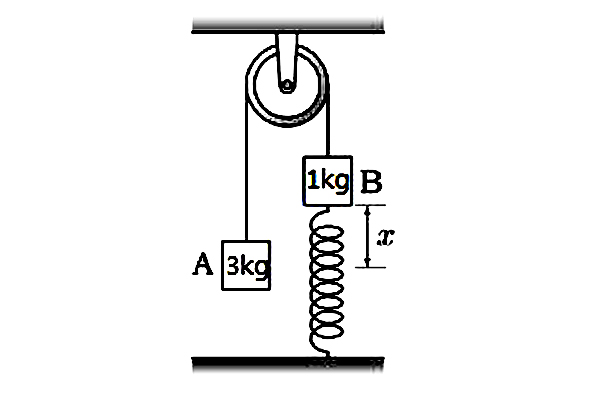# Spring constantThe system in the above diagram is static. Find $x$ (in cm), the extension of the spring, when the spring constant $k$ is $100 \text{ N/m}.$

Gravitational acceleration $g= 10 \text{ m/s}^2.$

×

Problem Loading...

Note Loading...

Set Loading...